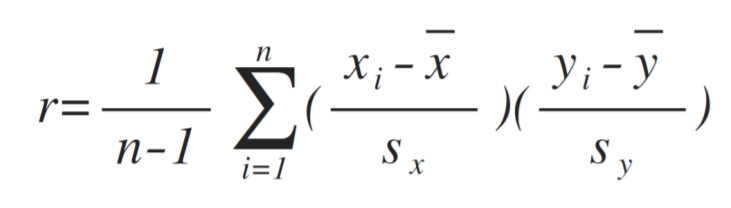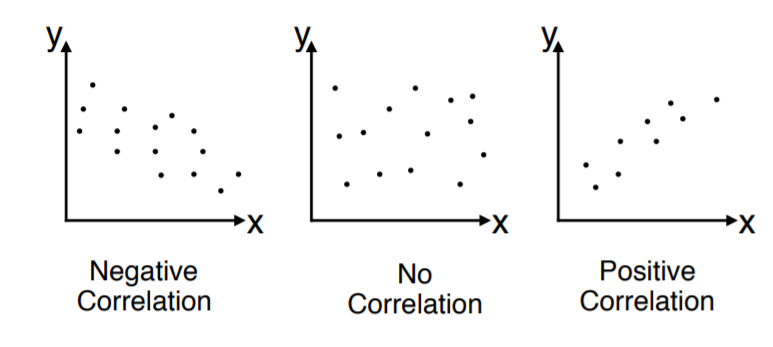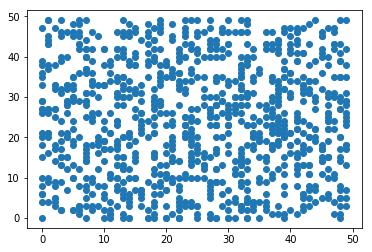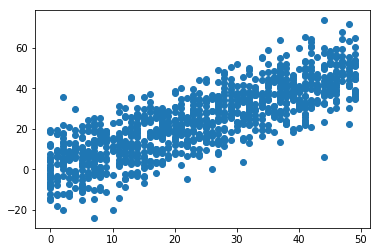#DAY 27
0

## ML_Day27(相關係數簡介)• 弱相關
``````import numpy as np
import matplotlib
import matplotlib.pyplot as plt

np.random.seed(1)
x = np.random.randint(0, 50, 1000)
y = np.random.randint(0, 50, 1000)
np.corrcoef(x, y)
plt.scatter(x, y)
``````• 正相關
``````x = np.random.randint(0, 50, 1000)
y = x + np.random.normal(0, 10, 1000)
np.corrcoef(x, y)
plt.scatter(x, y)
``````